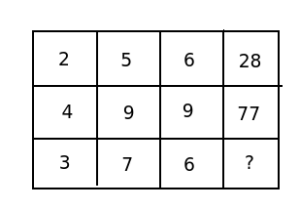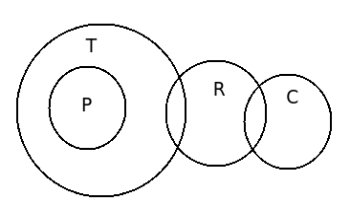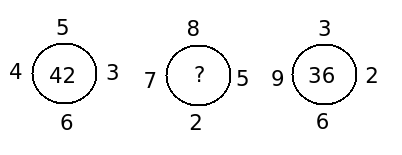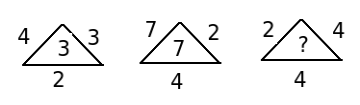# Logical reasoning - Quantitative Aptitude (MCQ) questions

Dear Readers, Welcome to Quantitative Aptitude Logical reasoning questions and answers with explanation. These Logical reasoning solved examples with shortcuts and tricks will help you learn and practice for your Placement Test and competitive exams like Bank PO, IBPS PO, SBI PO, RRB PO, RBI Assistant, LIC,SSC, MBA - MAT, XAT, CAT, NMAT, UPSC, NET etc.

After practicing these tricky Logical reasoning multiple choice questions, you will be exam ready to deal with any objective type questions.

1)   Find the missing number- Published on 06 May 16

a. 40
b. 38
c. 39
d. 35
e. None of these
 Answer  Explanation ANSWER: 39 Explanation: We have :In first row, (5 * 6) – 2 = 30 – 2 = 28In second row, (9 * 9) – 4 = 81 – 4 = 77Thus,In third row, the missing number is (7 * 6) – 3 = 42 – 3 = 39

2)   If “*” is called “+”, “/” is called “*”, “-” is called  “/”, “+” is called “-”. 40/20 – 5 * 10 + 5 = ?
- Published on 06 May 16

a. 170
b. 160
c. 150
d. 175
e. None of these
 Answer  Explanation ANSWER: None of these Explanation: Given : 40/20 – 5 * 10 + 5 = ?Substituting the coded symbols for mathematical operations, we get,40 * 20/5 +10 – 5 = ?40 * 4 + 10 – 5 = ?160 + 10 – 5 = ?170 – 5 = 165

3)   If Reena says, “Anjali's father Raman is the only son of my father-in-law Ramanand”, then how is Piyu, who is the sister of Anjali, related to Ramanand ?
- Published on 06 May 16

a. Wife
b. Sister
c. Grand-daughter
d. Daughter
e. None of these
 Answer  Explanation ANSWER: Grand-daughter Explanation: Only son of Reena's father-in-law Ramanand is Reena's husband. So, Raman is Reena's husband and Anjali & Piyu are his daughter's, Thus, Piyu is Grand-daughter of Ramanand.

4)   How many 8's are present in the following sequence of numbers which are exactly divisible by both its preceding and following numbers ?

3  8  6  8  8  7  6  8  3  4  8  2  5  6  2  8  2  4  8  6  3  7  4  8  4  5  8  4

- Published on 06 May 16

a. 5
b. 6
c. 2
d. Less than 4
e. None of these
 Answer  Explanation ANSWER: Less than 4 Explanation: 3 8 6 8 8 7 6 8 3 4 8 2 5 6 2 8 2 4 8 6 3 7 4 8 4 5 8 4 We want a group of 3 numbers with “8” at the centre such that it would be exactly divisible by both its preceding and following numbers. There are 3 such groups. (See the underlined groups above).

5)   Assume that there are three statements followed by several conclusions. You have to take the given statements to be true, even if they seem to be at variance from commonly-known facts and then decide which of the given conclusion/s logically follows.

Statements :  I. All Pens are Tables
II. Some tables are rulers.
III. Some rulers are chalk.

Conclusion :  I. Some tables are chalk.
II. Some pens are chalk.
III. Some rulers are pens.
IV. Some chalk are rulers

- Published on 05 May 16

a. Only IV follows
b. Only II and I follows.
c. Only III follows.
d. Only I, II and III follows
e. None of these
 Answer  Explanation ANSWER: Only IV follows Explanation:This diagram tells us that only one condition follows i.e. some chalk are rulers.

6)   Choose the number pair/group which is different from others.

1. 40 : 50
2. 30 : 36
3. 8 : 10
4. 28 : 35
5. 16 : 20

- Published on 05 May 16

a. 8 : 10
b. 40 : 50
c. 30 : 36
d. 28 : 35
e. 16 : 20
 Answer  Explanation ANSWER: 30 : 36 Explanation: 40 : 50 ==> 4 : 530 : 36 ==> 5 : 68 : 10 ==> 4 : 528 : 35 ==> 4 : 516 : 20 ==> 4 : 5In each of the pair except 30 : 36, the ratio of the two numbers is 4 : 5.

7)   Arrange the given words in the sequence in which they occur in the dictionary and choose the correct sequence.

1. Economic
2. Earlier
3. Each
4. Edition
5. Eager

- Published on 05 May 16

a. 3, 4, 1, 5, 2
b. 2, 1, 3, 5, 4
c. 3, 2, 4, 1, 5
d. 3, 5, 2, 1, 4
e. None of these
 Answer  Explanation ANSWER: 3, 5, 2, 1, 4 Explanation: The correct alphabetical order is Each, Eager, Earlier, Economic, Edition

8)   Find the missing number- Published on 05 May 16

a. 50
b. 51
c. 48
d. 53
e. 55
 Answer  Explanation ANSWER: 51 Explanation: We have :(5 * 6) + (4 * 3) = 30 + 12 = 42(3 * 6) + (9 * 2) = 18 + 18 = 36Therefore, the missing number is (8 * 2) + (7 * 5) = 16 + 35 = 51

9)   Arrange the following in a meaningful sequence :

1. Letter
2. Sentence
3. Word
4. Phrase

- Published on 05 May 16

a. 1, 2, 4, 3
b. 2, 1, 3, 4
c. 2, 4, 1, 3
d. 1, 3, 4, 2
e. None of these
 Answer  Explanation ANSWER: 1, 3, 4, 2 Explanation: A group of letters makes a word. A group of words makes a phrase. A group of phrases makes a sentence.Thus, correct order is 1, 3, 4, 2.

10)   Find the missing number- Published on 04 May 16

a. 5
b. 8
c. 4
d. 2
e. None of these
 Answer  Explanation ANSWER: 4 Explanation: We have :4 * 3 * 2 / 8 = 24/8 = 37 * 2 * 4 / 8 = 56/8 = 7Thus, missing number = 2 * 4 * 4/8 = 32/8 = 4

1 2 3 4